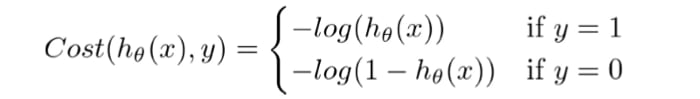Home Machine Learning Logistic Regression and its Cost Function

# Logistic Regression and its Cost Function [Detailed Introduction]

In this post, we are going to discover in-depth the Logistic Regression and its cost function.

Logistic Regression is a statistical machine learning model mainly used for binary classification problems. It is used when the target variable is categorical. Logistic regression can be used to classify –

1. Whether an email is spam or not
2. Whether a tumor is malignant or not.

The logistic regression can also be used for multiclass classifications too, and it is known as multinomial logistic regression, but in this be post we are going to limit ourselves to Binary Logistic Regression.

### Logistic Regression Representation

Logistic Regression uses probabilities to classify the data. It’s similar to linear regression, except it uses sigmoid function instead of the linear function. Hence the plot created is S-shaped instead of a straight line.

`Logistic Regression  =  Linear Regression + Sigmoid function`

For, Linear Regression, Z = WX + B

For, Logistic Regression, (hypothesis function) hΘ(x) = sigmoid (Z),
since the output of sigmoid function lies in the range [0,1], hence the logistic regression always results in values lying in the range [0,1].

If Z approaches +ve infinity, the predicted value becomes 1, and if Z approaches -ve infinity, the predicted value becomes 0.

### Cost Function for Logistic Regression

Cost Function/ Error function tells us how good is our model at making predictions for the given dataset. We try to minimize cost function in order to develop an accurate model with minimum error. In linear regression, we use mean square error, which results in the convex cost function, but if we use mean square error in logistic regression, the cost function will end up being non-convex, with many local minima, and in such cases, gradient descent (may) fail to optimize cost function properly as it may end up choosing any local minima as global minima instead of the actual global minima.

Hence in the case of logistic regression, we cannot use the mean square error function.

For logistic regression, the cost function is defined in such a way that it preserves the convex nature of loss function. The cost/loss function is divided into two cases: y = 1 and y = 0.After, combining them into one function, the new cost function we get is –

The above loss function is convex which means that it has a single minimum and the network won’t be stuck in local minimum(s) which are present in non-convex loss functions.

Now, we have got the cost function, but still, we have to minimize the cost value to get a more accurate classifier. For minimizing the cost function we can either use gradient descent or Newton method and both the methods require calculating the derivative of the above loss function.

### The derivative of the Cost Function

The weight matrix W and the biases matrix B are randomly initialized. We will use gradient descent to optimize(minimize) the cost function and then update the weights and biases to find the best fitting curve.

Since Logistic Regression uses a sigmoid function, so let’s start by finding the derivative of the actual sigmoid function itself.

Similarly, we can calculate the gradient for bias(B) also, which will be the same as above except the x(input vector) will not be present in the product term w. The resulting function will look something like this.

Let’s plug these values back in the cost function and multiply it with the learning rate(alpha) and update the Weights(W) and Biases(B). These equations are for a single epoch, we have to run multiple epochs and update the values of W & B until we don’t reach closer to the global minima.

But how will updating Weights(W) and Bias(B) make my result more accurate and reduce errors?

As we saw at the beginning of the post, we have drawn an arbitrary S-shaped line, but we don’t know whether that line does the most accurate classification. Perhaps, if the same S-Shaped line drawn differently might be possible we get more accurate results and fewer errors. Hence we constantly update the slope(W) and the y-intercept(B) of the arbitrary line to find out which straight line that will give us fewer errors and better classification results.  Note, by updating the W and B of a line, we are updating the slope and y-intercept of a curve.